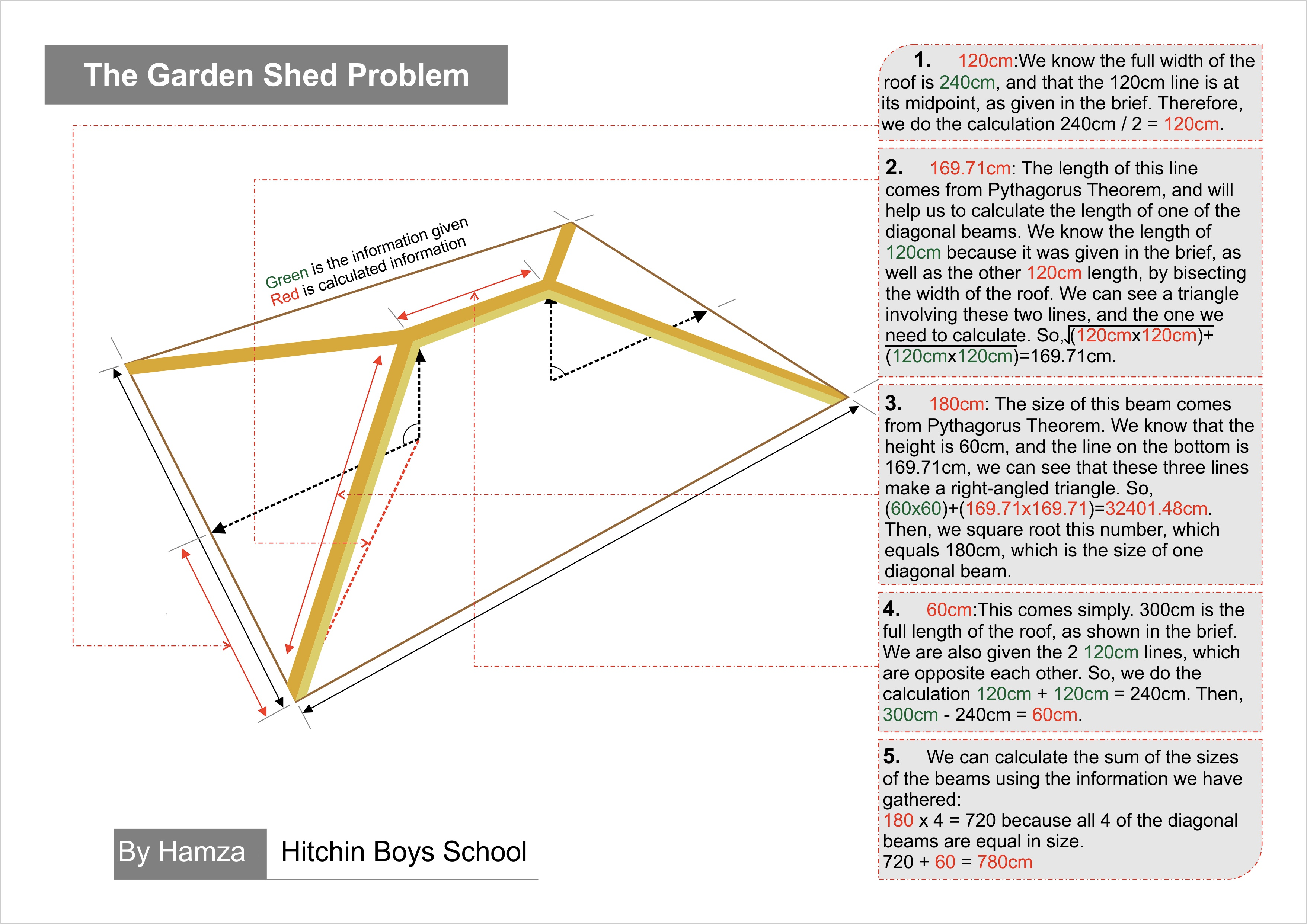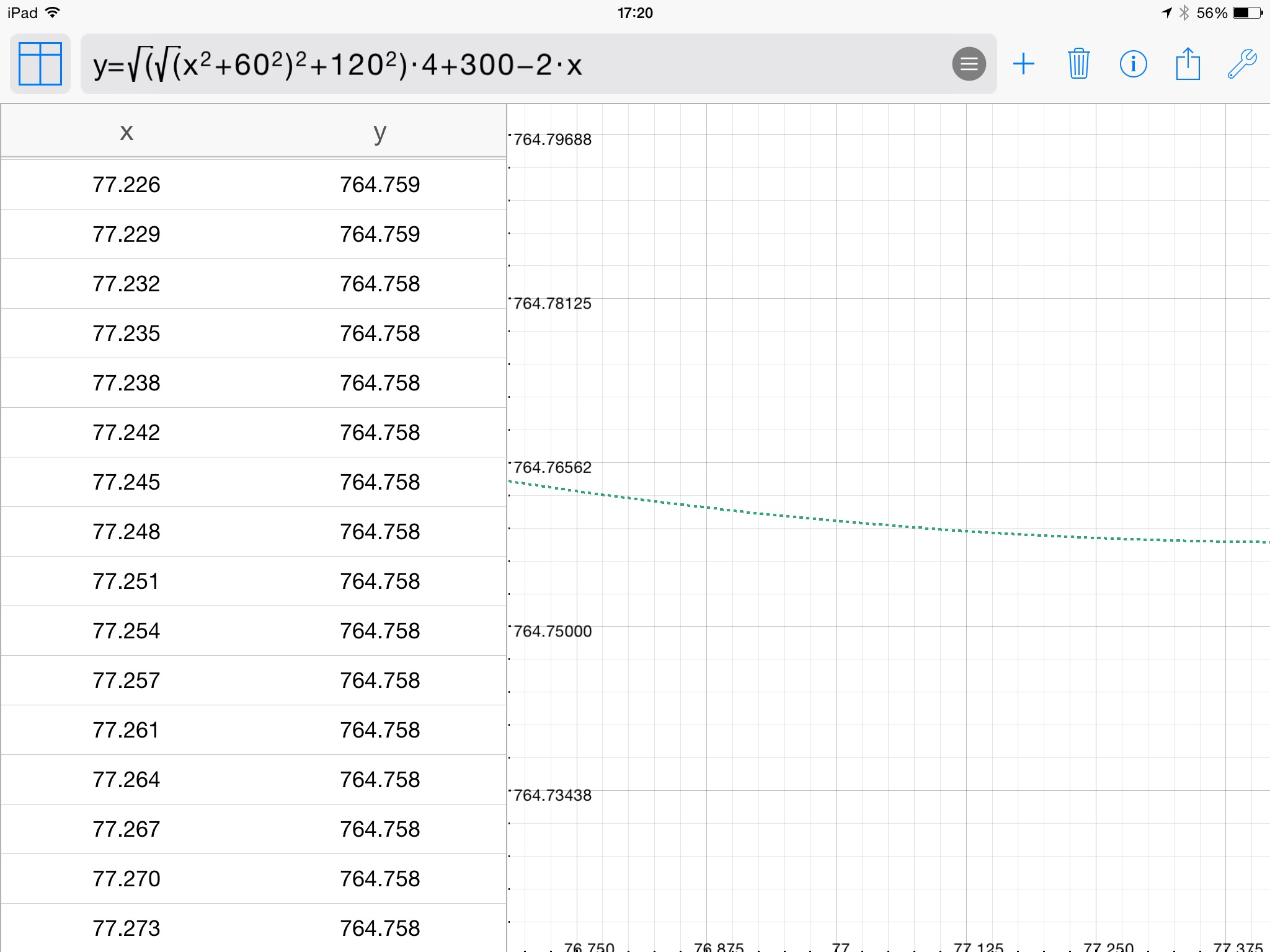#### You may also like### Square Pegs

Which is a better fit, a square peg in a round hole or a round peg in a square hole?### The Old Goats

A rectangular field has two posts with a ring on top of each post. There are two quarrelsome goats and plenty of ropes which you can tie to their collars. How can you secure them so they can't fight each other but can reach every corner of the field?### Isosceles

Prove that a triangle with sides of length 5, 5 and 6 has the same area as a triangle with sides of length 5, 5 and 8. Find other pairs of non-congruent isosceles triangles which have equal areas.

# Garden Shed

##### Age 11 to 14Challenge Level
Together, the beams of wood used to make the shed roof measure $780cm$. Here's a really clear solution diagram that Hamza from Hitchin Boys' School made:The second part of the question asked whether we could use less wood by changing the red length of $120cm$. Lots of people who thought about this question used different methods.

Sean from Sacred Heart Catholic College tried some different values for this red length to see if they used less wood:

If the red length was $100cm$ the middle beam would be $100cm$ also, we can tell this using the bird's eye view.

Then, using the same technique as the first part of the question with triangles and Pythagoras' theorem I discovered the sloping beams are $167.3cm$.

So a roof with red length $100cm$ would require $4x167.3+100=769.2cm$ of
wood; $10.8cm$ less than that needed for a roof with red length $120cm$.

Eleanor thought about the length of wood needed as a function of the red length, and drew a graph of this function:

Let the length of the red line be $x$. To find the shortest possible length, the best way is to plot a graph of the points for the equation $y=\sqrt{x ²+18000} \times 4 + 300-2x$. This is the total length of wood needed when the red length is $x$. I used an app to plot my graph and give me the table, screenshots below. The table of results shows that the shortest possible legnth of wood need is $764.758$ and the value of $x$ needed for this is approximately $77.5cm$.Joshua from QEGS Penrith and Niharika from Rugby School also thought about the total length of wood needed as a function of the red length, and used calculus to find the minimum value this function takes. Here is a link to Niharika's solution.